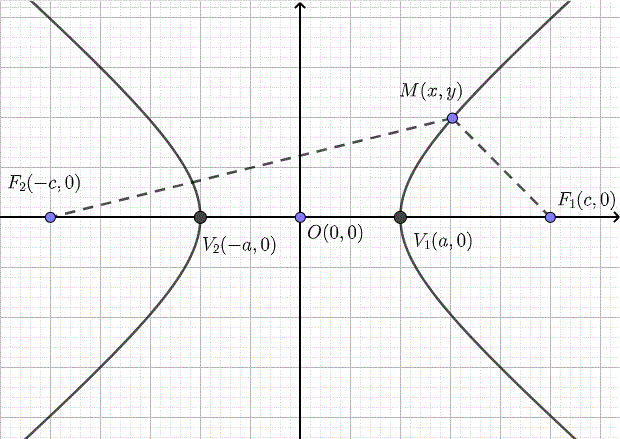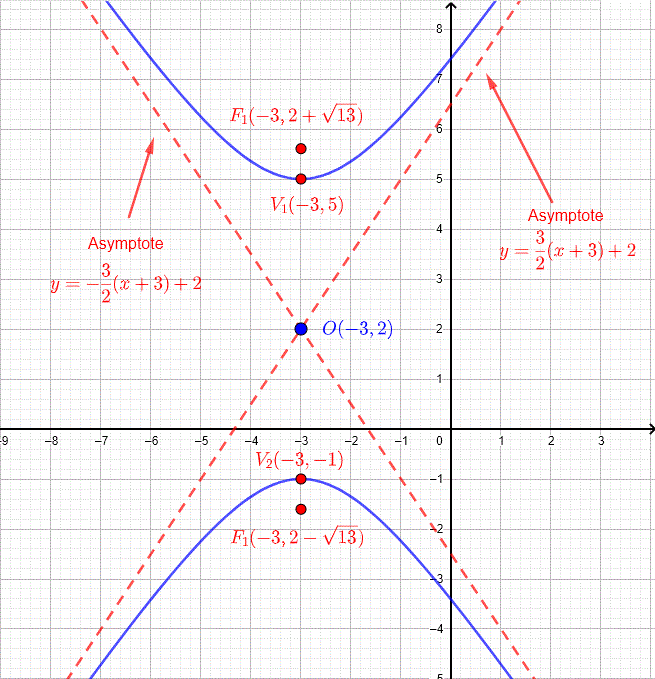# Equation of Hyperbola

## Definition and Equation of a Hyperbola with Horizontal Transverse Axis

A yperbola is the set of all points $M(x,y)$ in a plane such that the difference of the distances from $M$ to fixed points $F_1$ and $F_2$ called the foci (plurial of focus) is equal to a constant.
$\overline{MF_2} - \overline{MF_1} = \sqrt{(x+c)^2+y^2} - \sqrt{(x-c)^2+y^2} = \pm 2 a$
Eliminating the square roots by squaring and simplifying using the relationship $c^2 = a^2 + b^2$, the standard equation of a hyperbola may be written as:

$\dfrac{x^2}{a^2} - \dfrac{y^2}{b^2} = 1$

where $a \gt 0$, $b \gt 0$ and $c \gt 0$.
Point $O(0,0)$ is the center of the hyperbola.
Points $V_1(a,0)$ and $V_2(-a,0)$ are called the vertices of the ellipse.
The foci are at $F_1(c,0)$ and $F_2(-c,0)$Asymptotes of Hyperbola
If we solve the equation of the hyperbola for $y$, we obtain
$y = \pm \dfrac{b}{a} \sqrt{x^2 - a^2}$
As $|x|$ increases indefinitely, $y$ approaches $\pm \dfrac{b}{a} x$. Hence the lines with equations $y = \pm \dfrac{b}{a} x$ are called asymptotes of the hyperbola.
The graph of a hyperbola and its asymptotes (broken lines) are shown below.Example 1
A hyperbola, with horizontal transverse axis , centered at $(0,0)$ has x intercepts at $(3,0)$ and $( -3 ,0)$ and foci at $(5,0)$ and $(-5,0)$. Find the equation of the hyperbola and its asymptotes.

Solution to Example 1
The x intercepts of a hyperbola with horizontal transverse axis are the vertices given by $(a , 0)$ and $(-a , 0)$ . Hence $a = 3$
The foci are given by $F_1(c,0) = F_1(5,0)$ and $F_2(-c,0) = F_2(-5,0)$. Hence $c = 5$
$a$, $b$ and $c$ are related by $c^2 = a^2 + b^2$ which gives the equation
$5^2 = 3^2 + b^2$
Solve to obtain
$b = 4$
The equation of the hyperbola is given by
$\dfrac{x^2}{3^2} - \dfrac{y^2}{4^4} = 1$

General Equation
We can generalize and write the equation of a hyperbola whose center is at $O(h,k)$ as follows

$\dfrac{(x-h)^2}{a^2} - \dfrac{(y-k)^2}{b^2} = 1$

with foci at $F_1(c+h,k)$ and $F_2(-c+h,k)$ and vertices at $V_1(a+h,k)$ and $V_2(-a+h,k)$, and the relationship $c^2 = a^2 + b^2$.
The asymptotes are given by $y = \pm \dfrac{b}{a} (x - h) + k$

Example 2 Find the equation of the hyperbola with vertices at $V_1(2,2)$ and $V_2(-4,2)$ and whose asymptotes are given by the equations $y = \pm \dfrac{2}{5} (x - h) + k_0$ where K_0 is a constant. Find the equations of the asymptotes and plot the graph of the given hyperbola and its asymptotes.

Solution to Example 2
The vertices are given by $V_1(a+h,k) = V_1(2,2)$ and $V_2(-a+h,k) = V_2(-4,2)$
which gives $k = 2$ and $a+h = 2$ and $-a+h = - 4$
Solve the last two equations in $a$ and $h$ to obtain
$a = 3$ and $h = -1$
The asymptotes are given by $y = = \pm \dfrac{2}{5} (x - h) + K_0 = \pm \dfrac{b}{a} (x - h) + k$
Hence $\dfrac{b}{a} = \dfrac{2}{5}$
Substitute $a$ by $3$ in the above equation and solve for $b$ to obtain
$b = \dfrac{6}{5}$
$K_0 = k = 2$
The equations of the asymptotes are given by $y = = \pm \dfrac{2}{5} (x - h) + 2$## Equation of a Hyperbola with Vertical Transverse Axis

The equation of a hyperbola with Vertical Transverse Axis whose center is at $O(h,k)$ is given by

$\dfrac{(y-k)^2}{a^2} - \dfrac{(x-h)^2}{b^2} = 1$

with foci at $F_1(h,k+c)$ and $F_2(h,k-c)$ and vertices at $V_1(h,k+a)$ and $V_2(h,k-a)$, and the relationship $c^2 = a^2 + b^2$.
The asymptotes are given by $y = \pm \dfrac{a}{b} (x - h) + k$

Example 3
The equation of a hyperbola is given by $\dfrac{(y-2)^2}{3^2} - \dfrac{(x+3)^2}{2^2} = 1$. Find its center, foci, vertices and asymptotes and graph it.

Solution to Example 3
The given equation is that of hyperbola with a vertical transverse axis. Compare it to the general equation given above, we can write
$h = -3, k = 2, a = 3$ and $b = 2, c = \sqrt{a^2 + b^2} = \sqrt{3^2 + 2^2} = \sqrt{13}$
The center is at $O(h,k) = O(-3,2)$
The foci are at $F_1(h,k+c) = F_1(-3,2+\sqrt{13})$ and $F_2(-3,2-\sqrt{13})$
The vertices are at $V_1(h,k+a) = V_1(-3,5)$ and $V_2(h,k-a) = V_2(-3,-1)$
The equations of the asymptotes are given by
$y = \dfrac{3}{2}(x + 3) + 2$ and $y = - \dfrac{3}{2}(x + 3) + 2$
The graph of the given equation of the hyperbola, its center, foci, vertices and asymptotes is shown below.## Interactive Tutorial on Equation of a Hyperbola with Horizontal Transverse Axis

An app to explore the equation of a hyperbola and its properties is now presented. The equation used is the standard equation that has the form

$\dfrac{(x - h)^2}{a^2} - \dfrac{(y - k)^2}{b^2} = 1$

The exploration is carried out by changing the parameters $a, b, h$ and $k$ included in the above equation of the hyperbola. The default values, when you open this page, are: $a = 2, b = 3, h = -1$ and $k = 2$.
Some activities are listed below but many other activities may be generated.
Click on the button "Plot Equation" to start.

 $a$ = 2 $b$ = 3 $h$ = -1 $k$ = 2

Hover the mousse cursor on the graph or plotted point to read the coordinates.

Click the button above "Plot Equation". You may hover the mousse cursor over the graph to trace the coordinates. You may also Hover the mousse cursor on the top right of the graph to have the option to download the graph as a png file.
1 - Select a point M on the graph of the hyperbola, hover the mousse over it and read the coordinates. Read the coordinates of F1 and F2 (right legend) or hover the mousse and read their coordinates and show that the difference of the distances $\overline{MF_1} - \overline{MF_2}$ is close to $2a$ if $\overline{MF_1} \gt \overline{MF_2}$ or $- 2a$ if $\overline{MF_1} \lt \overline{MF_2}$.
You may do the above activity for different values of $a, b, h$ and $k$ with as many points as needed to better understand the definition of an hyperbola.
2 - Use the values of $a$ and $b$ to find $c$ using the relationship between $a, b$ and $c$ given by $c^2 = a^2 + b^2$.
Use the above results to find the coordinates of $F_1, F_2, V_1$ and $V_2$ and check the results graphically.

3 - Set $a, b, h$ and $k$ to some values. Find the x and y intercepts and check your results graphically.
4 -
Exercise:
Show by algebraic calculations that the following equation $\dfrac{(x + 2)^2}{5} - 5(y-3)^2 = 5$ is that of a hyperbola and find the center, foci and vertices of the ellipse given by the equation then use the app to graph it and check your answers.

If needed, Free graph paper is available.

## More References and Links to Topics Related to the Equation of the Hyperbola

Hyperbola
Equation of Hyperbola- Graphing Problems
College Algebra Problems With Answers sample 10 : Equation of Hyperbola
Similar tutorials on
circle , Ellipse and Parabola